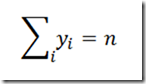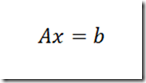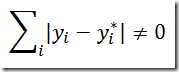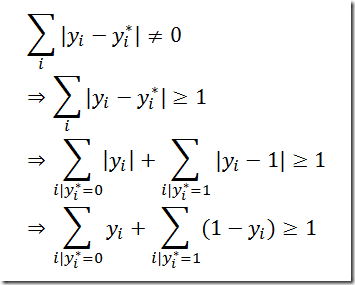## Monday, October 31, 2011

### Slacks (with penalties) should not be integer variables

In the paper http://www.cs.nott.ac.uk/~jxm/timetabling/gor2007-paper.pdf a number of integer models for timetabling are discussed. The author also published the models used in this paper http://www.cs.nott.ac.uk/~jxm/timetabling/gor2007-empirical.zip (this should be very much applauded). Of course that means that I can provide some criticism.

In the scheduling models some soft constraints are modeled by introducing slacks, in this case CourseMinDayViolations:

 subto ctCountWorkingDays:   forall in Courses:   sum in Days:   CourseSchedule[c,d] >= CourseHasMindays[c] - CourseMinDayViolations[c];

This variable can then be minimized in the objective:

 minimize value:     (sum

in Periods: sum in Rooms: sum in Courses with CourseHasStudents[c] > RoomHasCapacity[r]:       (Taught[p,r,c] * (CourseHasStudents[c] - RoomHasCapacity[r]))     )   + 2 * (sum in Curricula: sum in Days: sum in DaySingletonChecks: SingletonChecks[cu,d,sc])   + 5 * (sum in Courses: CourseMinDayViolations[c]) ;

That looks all good. But I don’t agree with the declaration:

 var CourseMinDayViolations[Courses] integer >= 0 <= nbDays;

A slack variable like this should be declared as a positive continuous variable. It will be integer automatically. With this declaration there is the possibility the solver will branch on this variable.

My simplest argument would be: a model with fewer integer variables is likely to solve faster.

But sometimes solvers actually add integer variables! See:

 Tried aggregator 1 time. MIP Presolve eliminated 1634 rows and 5 columns. MIP Presolve modified 10 coefficients. Reduced MIP has 2401 rows, 11512 columns, and 92222 nonzeros. Reduced MIP has 11028 binaries, 0 generals, 0 SOSs, and 0 indicators. Probing time =    0.02 sec. Tried aggregator 1 time. MIP Presolve eliminated 10 rows and 0 columns. Reduced MIP has 2391 rows, 11512 columns, and 91732 nonzeros. Reduced MIP has 11032 binaries, 480 generals, 0 SOSs, and 0 indicators. Presolve time =    0.20 sec.

(This model had originally only binary and continuous variables).

## Wednesday, October 26, 2011

### Still not proven optimal…

This is a very small MIP:

 Starting Gurobi... Optimize a model with 24 rows, 54 columns and 132 nonzeros Presolve removed 6 rows and 6 columns Presolve time: 0.11s Presolved: 18 rows, 48 columns, 114 nonzeros Variable types: 12 continuous, 36 integer (0 binary)

so I expected to run this very quickly on a fast 8 core Xeon machine. The time limit of 9999 seconds should be no problem even with the OPTCR (rel. gap tolerance) of 0.0. But I see:

 835689342 7572026 infeasible   63         0.00062    0.00000   100%   1.0 9980s 836077602 7576642    0.00000   65   14    0.00062    0.00000   100%   1.0 9985s 836454289 7582365    0.00000   56   15    0.00062    0.00000   100%   1.0 9990s 836850333 7587882    0.00000   45   15    0.00062    0.00000   100%   1.0 9995s Cutting planes:   Gomory: 1   MIR: 2 Explored 837155963 nodes (841647794 simplex iterations) in 9999.00 seconds Thread count was 8 (of 8 available processors) Time limit reached Best objective 6.179540598832e-04, best bound 0.000000000000e+00, gap 100.0000% MIP status(9): Optimization terminated due to time limit.

This is not a Gurobi problem: Cplex is just as bad. Apparently these branch and bound methods are just not suited very well for this problem. I have solved some huge problems with hundreds of thousands of binary variables, but here we see some evidence, not all problems are that easy.

Recently I saw someone writing:

You may all drool now.

Guess we need something like that to solve my tiny 36 integer variable problem….

Notes:

• The OPTCR (gap tolerance) setting is not an issue as it turns out the best bound remains at 0.0, so a gap of 100% is reported.
• Gurobi is solving the nodes pretty efficiently. Approx. 1 simplex iteration per node, and > 80k nodes per second.

#### Update

Some Cplex runs solve this fast (see comments below), but on some machines I see the same problem:

 Cplex 12.3.0.0 Reading data... Starting Cplex... Tried aggregator 2 times. MIP Presolve eliminated 1 rows and 1 columns. MIP Presolve modified 36 coefficients. Aggregator did 6 substitutions. Reduced MIP has 18 rows, 48 columns, and 114 nonzeros. Reduced MIP has 0 binaries, 36 generals, 0 SOSs, and 0 indicators. … Elapsed real time = 9880.28 sec. (tree size = 1251.36 MB, solutions = 70) Nodefile size = 1123.85 MB (527.18 MB after compression) 478309344 6481362        0.0000    15        0.0012        0.0000 5.26e+008  100.00% 479049990 6483695        0.0000    15        0.0012        0.0000 5.27e+008  100.00% 479749224 6499596        0.0000    15        0.0012        0.0000 5.27e+008  100.00% 480524806 6502892    infeasible              0.0012        0.0000 5.28e+008  100.00% 481284148 6500253        0.0000    16        0.0012        0.0000 5.29e+008  100.00% 482022525 6501343        0.0000    16        0.0012        0.0000 5.30e+008  100.00% 482764367 6511470        0.0000    16        0.0012        0.0000 5.31e+008  100.00% Mixed integer rounding cuts applied:  2 Zero-half cuts applied:  2 Gomory fractional cuts applied:  2 Root node processing (before b&c):   Real time             =    0.03 Parallel b&c, 4 threads:   Real time             = 9998.98   Sync time (average)   =  420.45   Wait time (average)   =   45.69                           ------- Total (root+branch&cut) = 9999.01 sec. MIP status(107): time limit exceeded Fixing integer variables, and solving final LP... Parallel mode: deterministic, using up to 2 threads for concurrent optimization. Tried aggregator 1 time. LP Presolve eliminated 25 rows and 55 columns. All rows and columns eliminated. Fixed MIP status(1): optimal Resource limit exceeded. MIP Solution:            0.001230    (530937464 iterations, 482992718 nodes) Final Solve:             0.001230    (0 iterations) Best possible:           0.000000 Absolute gap:            0.001230 Relative gap:            1.000000

This is a worse solution than Gurobi found. But on a different machine using 8 threads:

 Cplex 12.3.0.0 Reading data... Starting Cplex... Tried aggregator 2 times. MIP Presolve eliminated 1 rows and 1 columns. MIP Presolve modified 36 coefficients. Aggregator did 6 substitutions. Reduced MIP has 18 rows, 48 columns, and 114 nonzeros. Reduced MIP has 0 binaries, 36 generals, 0 SOSs, and 0 indicators. … 129959  4046        0.0003    10        0.0031        0.0003   113627   91.54% *165794  5594      integral     0        0.0027        0.0005   146606   81.09% *170030  4859      integral     0        0.0019        0.0005   149634   72.63% *193953  3702      integral     0        0.0007        0.0006   171409   15.85% *195840   418      integral     0        0.0006        0.0006   172394    2.03% Mixed integer rounding cuts applied:  3 Gomory fractional cuts applied:  1 Root node processing (before b&c):   Real time             =    0.12 Parallel b&c, 8 threads:   Real time             =    4.10   Sync time (average)   =    0.39   Wait time (average)   =    0.02                           ------- Total (root+branch&cut) =    4.23 sec. MIP status(101): integer optimal solution Fixing integer variables, and solving final LP... Parallel mode: deterministic, using up to 2 threads for concurrent optimization. Tried aggregator 1 time. LP Presolve eliminated 25 rows and 55 columns. All rows and columns eliminated. Fixed MIP status(1): optimal Proven optimal solution. MIP Solution:            0.000616    (175023 iterations, 203294 nodes) Final Solve:             0.000616    (0 iterations) Best possible:           0.000616 Absolute gap:            0.000000 Relative gap:            0.000000

So we have some very different behavior here: optimal in just 4 seconds.

## Thursday, October 20, 2011

### Special case of integer cuts

In http://yetanothermathprogrammingconsultant.blogspot.com/2011/10/integer-cuts.html we derive a constraint that forbids an integer configuration y*.

If the model contains a constraint like:then we can simplify the integer cut to:as we only need to keep track of the variables flipping from 1 → 0. This is because any variable moving from 0 → 1 will cause another variable to move from 1 → 0.

This simpler constraint has fewer nonzeroes which is important if we add a lot of such cuts, as is the case in one of my models.

## Tuesday, October 18, 2011

### MPS files

Yesterday we found out that Microsoft Solver Foundation is writing incorrect MPS files when the model has general integer variables without an upper bound. See: http://social.msdn.microsoft.com/Forums/en-US/solverfoundation/thread/7669081f-18ac-445b-850e-434bff80da38.

Right after I submitted an error report, the following e-mail arrived:

 I trying to convert one problem on gmpl format to free mps format, both in attached, and the solve it. Unfortunately, when solve the mps file I get an answer different from the answer that I get when solve using the gmpl file. I was using the following commands: \$ glpsol --math exemp01.mod \$ glpsol --math exemp01.mod --check --wfreemps exemp01.mps \$ glpsol --freemps exemp01.mps

Lo and behold, this is exactly the same problem!

The problem is shown in the following MPS file (generated by Cplex, comments added by me):

 NAME          gamsmodel ROWS N  obj E  e1 L  e2 COLUMNS     MARK0000  'MARKER'                 'INTORG'     x         obj                            -1     x         e2                              1     MARK0001  'MARKER'                 'INTEND'     z         obj                            -1     z         e1                              1 RHS     rhs       e2                            200 BOUNDS * column x is a general integer variable * it needs an LI card to make sure it is not * interpreted as a binary variable LI bnd       x         0 FR bnd       z ENDATA

This LI card is missing from the GLPSOL and Solver Foundation generated files. The rule is: if no bounds are specified, an integer variable has default bounds 0 and 1. Obviously this will change the meaning of the model.

Note: In GAMS by default all integer variables have a finite upper bound, so no problem there. It is possible (with some effort) to create a model with +INF bounds on integer variables (using PF4=0 command line option). It turns out that with such a model, the tool CONVERT (this tool can write MPS files), produces an MPS file with the same error. Admittedly somewhat contrived, but not correct anyway.

## Sunday, October 16, 2011

### Sorting by MIP

Here is a toy model that illustrates that certain models are poorly suited for solving by MIP solvers.

Sorting data is a rather elementary operation. Surprisingly, GAMS has nothing built in for this. There is an external tool for sorting (gdxrank), but in some environments (e.g. NEOS) this is not allowed. There are some slower GAMS coding tricks that can help. Of course we can also write a simple MIP model that performs sorting:

 \$ontext    mip model for sorting \$offtext set i /i1*i100/; parameter p(i) "original data"; p(i) = uniform(0,1); alias (i,j); binary variable x(i,j) "permutation matrix"; variable y(i) "sorted version of p"; variable z; equations   dummy    "dummy objective"   assign1  "assignment constraint"   assign2  "assignment constraint"   ydef     "y is permuted version of x"   sort     "require y to be sorted" ; dummy.. z =e= 0; assign1(i).. sum(j, x(i,j)) =e= 1; assign2(j).. sum(i, x(i,j)) =e= 1; ydef(i)..    y(i) =e= sum(j, x(i,j)*p(j)); sort(i+1)..  y(i+1) =g= y(i); model m /all/; solve m minimizing z using mip; display   "-----------------------------------------------------------------------",   "Results",   "-----------------------------------------------------------------------",    p,y.l;

This is quite slow however. n=100 is no problem, but n>200 will require significant time (>1000 seconds). Constraints assign1 and assign2 form an assignment problem, which is easy to solve. The simple extension will cause relaxation based MIP solvers to slow down enormously. Obvious question: are there better formulations? I would guess constraint programming solvers would do better on this.

For some instances Gurobi is doing very good, probably because of its excellent heuristics. But this is not the case for all instances: in some cases it is just as slow as other solvers.

The above model contains two noteworthy modeling tricks:

• a dummy objective so that we stop after the first integer solution is found
• the equation sort is indexed over i+1 so that the last one is not generated: this equation has card(i)−1 members.

### MIQP: optimality tolerances vs objective scaling

I was working on a MIQP model in finance: the optimal allocation of contracts to client accounts so that each client observes an average price that is as close as possible to the average contract price. The model was implemented in Solver Foundation (for the client) and GAMS (for prototyping).

With one data set we observed Gurobi was giving slightly sub-optimal solutions. I.e. the obj was 1.396560E-5 instead of the number found by other solvers: 0. Most likely this is a tolerance problem. I tried the optimality tolerance (from 1e-6 to 1e-8 and 1e-9) but that did not help. That option is just for the LP/QP subproblems. What actually did the trick was multiplying the objective by a 1000:

 Goals[Minimize["Objective"->    1000*Sum[{a,accounts}, (PriceAlloc[a]-AvgPrice)^2] ]],

Apparently this is not scaled away (somewhat to my surprise), allowing us to convey the solver not to stop too early.

I also checked: reset the relative gap tolerance to 0 from its default of 1.0e-4. In the GAMS and Solver Foundation version of the model this did not help.

Update:

• On some new data sets we still see somewhat unstable behavior: different solutions with different objs by just reordering data.
• Gurobi solves KKT conditions, so may need to tighten feasibility tolerance. This helps somewhat, but not completely. Question: I suppose this introduces some danger of declaring models infeasible.
• I get better stability when formulated as a MIP (using an absolute value formulation).

## Tuesday, October 4, 2011

### Solving a large sparse system of equations with an LP solver

When we want to solve a large, sparse system of linear equationsusing an LP solver we expect this to be reasonably fast. After all, solving systems of equations is what an LP solver does all the time. So we introduce a zero objective and solve. With Gurobi a sample problem gives:

 Starting Gurobi... Optimize a model with 9801 rows, 9801 columns and 48609 nonzeros Presolve removed 4599 rows and 4599 columns Presolve time: 0.06s Presolved: 5202 rows, 5202 columns, 47242 nonzeros Iteration    Objective       Primal Inf.    Dual Inf.      Time        0      handle free variables                          0s     3958      handle free variables                          5s     4975      handle free variables                         11s     5199    0.0000000e+00   0.000000e+00   0.000000e+00     25s     5199    0.0000000e+00   0.000000e+00   0.000000e+00     25s Solved in 5199 iterations and 25.19 seconds

Actually we can much improve on this. By specifying an advanced basis. We know the equations (slacks) will be non-basic in the solution and all the variables will be basic. If we set up an advanced basis for this we see for the same problem:

 Starting Gurobi... Optimize a model with 9801 rows, 9801 columns and 48609 nonzeros Iteration    Objective       Primal Inf.    Dual Inf.      Time        0    0.0000000e+00   0.000000e+00   0.000000e+00      0s Solved in 0 iterations and 0.45 seconds

Indeed no simplex iterations were needed: just an invert.

### Update

In response to a comment about Octave: that is still faster:

 octave-3.2.4.exe:3> tmp=load("-ascii","mat.txt"); octave-3.2.4.exe:4> b=load("-ascii","rhs.txt"); octave-3.2.4.exe:5> a=sparse(tmp(:,1),tmp(:,2),tmp(:,3)); octave-3.2.4.exe:6> nnz(a) ans =  48609 octave-3.2.4.exe:7> tic();x=a\b;toc() Elapsed time is 0.057 seconds. octave-3.2.4.exe:8>

Notes:

• Not completely fair of course: the LP solver does still a lot of extra things (scaling, pricing etc.).
• Note that I used a sparse matrix in Octave. Not sure what method they use to solve this system.
• This was a structured test matrix: see: http://www.public.iastate.edu/~akmitra/aero361/design_web/Laplace.pdf. For more unstructured matrices Gurobi may do much better relative to Octave.

## Monday, October 3, 2011

### MIP caveats

In http://www.or-exchange.com/questions/3316/metrics-rules-of-thumb-tool-support-to-assess-mip-models a number of caveats when doing MIP modeling are listed:

1. All 0 objective: although it should be easier to find any solution than one specific, in certain situations it might pay off to add a fake objective function to guide the search. Anything reasonably stable should work and preferable all variables would have different objective coefficients. This sometimes works, sometimes not. I guess it also has to do with the fact that solvers usually detect this and do something about it.
2. Unstable objective: Very big and very small coefficients in the objective function, especially values below the dual tolerance, can cause issues with dual feasibility and very unexpected results.
3. One or few objective coeffcients: One can observe that MIP solvers have trouble solving instances with only one (or a few) non-zeros in the objective function. At least part of the problems with these models is dual degeneracy.

Point 1: I am not sure about this one. With Obj=0 we really want to stop as soon as a feasible integer solution is found. With an obj<>0 this is no longer the case (or am I misreading this; may be additionally the stopping criterion “stop after first feasible solution” is implied). I don’t have many MIPs without an objective (and first integer solution found is not always very good), so my experience is limited. Somehow I feel this would need more empirical evidence.

Point 2: agreed. E.g. with long planning horizons (like in forestry models) some of the reduced cost can become very small. Playing with LP optimality tolerances can help in such cases (I usually don’t want to touch those, but this is an exception).

Point 3: I don’t understand that completely. I have many MIPs with very few cost coefficients (often just one: e.g. max profit). I have seen cases where small “random” obj coefficients can help to break symmetry and force solutions to be different. But that is different than is described here.

I.e. in general these are not the problem areas I would quickly consider if I have a difficult MIP. Either this user has access to different solvers than I do or he is solving different types of models. Or both.

Anyway, it is interesting to see I have such a different experience about problem areas in MIP modeling. I also think that many “older” tricks (such as I mentioned under point 3, another one is using priorities) are more and more becoming obsolete due to smarter and more sophisticated MIP solvers. I have a few “old” models with some tuning tricks, that really nowadays solve faster without all the tricks.

## Sunday, October 2, 2011

### Ateji defunct: OptimJ now free

Unfortunately, the company Ateji has ceased to operate (http://www.ateji.com/blog/ateji-is-closed/, http://www.ateji.com/). They had an interesting modeling framework based on Java.

Sometimes I divide modeling tools up as follows:

1. Matrix generators: write LP or MPS file from application. This is done since the very early times. The main difference is that Fortran is no longer the obvious choice for programming language to use.
2. Specialized modeling languages: AMPL, GAMS, …
3. Low level solver APIs. This is essentially like writing a matrix generator, minus the part where you write a file. In many cases the solver API can help a little bit by providing routines like addrow() and addcol().
4. Hybrids, somewhere between 2 and 3, where modeling constructs are added to traditional programming languages. E.g. Cplex Concert, and OptimJ.

I suspect that OptimJ is mainly attractive for the intersection of the set of Java programmers and the set of modelers of optimization models. Besides that this subset may be somewhat small (many modelers are not proficient Java programmers), there is also a lot of competition targeting this particular group of users.

I believe that some of the more complex data issues in practical modeling are often better (i.e. more efficiently) dealt with in a specialized language than in a traditional programming language. In statistics this has largely been accepted. Much statistical work is done inside specialized “language” environments such as SAS, SPSS and Stata. In optimization this is not (yet) the case: the lower level APIs still have a large following. This while data is often more irregular in practical optimization than in statistics. Of course much statistical computing is done “off-line” so the integration issues that plague modeling languages are just not there.

### Integer cuts

I received a few questions about how to form constraints that exclude a known 0-1 integer solution. Assume our solution we want to exclude is called y* then we can write:This can be linearized as follows:The final result is also sometimes written as the following constraint:Note that y is a binary decision variable.